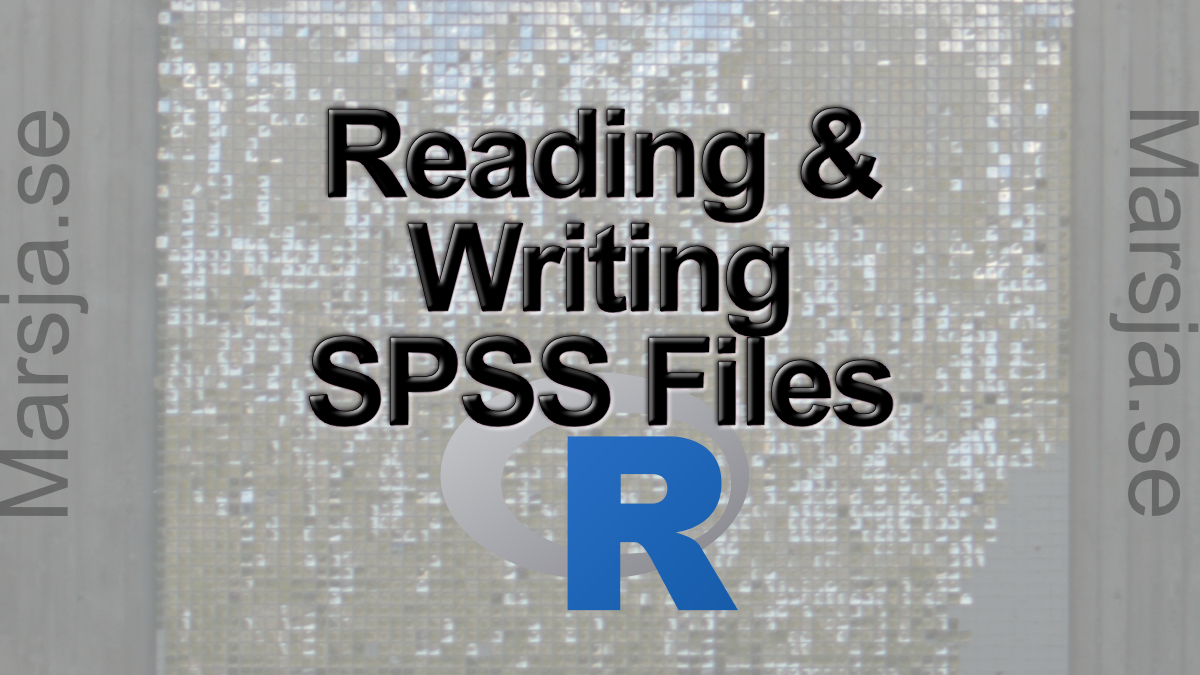15 Shares

In this post we are going to learn 1) how to read SPSS (.sav) files in R, and 2) how to write to SPSS (.sav) files using R.  More specifically, here we are going to work with the following two R packages haven (from the Tidyverse) and foreign to:

• Read a .sav file into an R dataframe
• Writing an R dataframe to a .sav file

## Data Import in R

R is a great statistical programming environment and we can store our data in the .rda format. However, sometimes we may collaborate with other researchers using other statistical software and/or that are storing their data in different formats. Luckily, as an R user we can import data from a range of different formats; SAS (.7bdat), Stata (.dta), Excel (e.g., .xlsx), and CSV (.csv) to name a few. Here are some tutorials about how to import data from some of these formats:

## Can R read SPSS files?

In this brief section, we are going to quickly answer the question about Can R read SPSS files? Short answer is yes! Here’s how:
1)`library(haven)`, and then
2) ` read_sav(PATH_TO_YOUR_FILE)`
Read the post for more details!

## How to Open an SPSS File in R

In this section, we are going to go into more detail on how to load a .sav file into an R dataframe. More specifically, we will start by using the R package haven and, then, continue using the foreign package.

### Importing a .sav file in R using Haven’s read_sav

In this section, we are going to learn how to load an SPSS file in R using the package Haven and the function read_sav.

```.wp-block-code {
border: 0;
}

.wp-block-code > span {
display: block;
overflow: auto;
}

.shcb-language {
border: 0;
clip: rect(1px, 1px, 1px, 1px);
-webkit-clip-path: inset(50%);
clip-path: inset(50%);
height: 1px;
margin: -1px;
overflow: hidden;
position: absolute;
width: 1px;
word-wrap: normal;
word-break: normal;
}

.hljs {
box-sizing: border-box;
}

.hljs.shcb-code-table {
display: table;
width: 100%;
}

.hljs.shcb-code-table > .shcb-loc {
color: inherit;
display: table-row;
width: 100%;
}

.hljs.shcb-code-table .shcb-loc > span {
display: table-cell;
}

.wp-block-code code.hljs:not(.shcb-wrap-lines) {
white-space: pre;
}

.wp-block-code code.hljs.shcb-wrap-lines {
white-space: pre-wrap;
}

.hljs.shcb-line-numbers {
border-spacing: 0;
counter-reset: line;
}

.hljs.shcb-line-numbers > .shcb-loc {
counter-increment: line;
}

.hljs.shcb-line-numbers .shcb-loc > span {
}

.hljs.shcb-line-numbers .shcb-loc::before {
border-right: 1px solid #ddd;
content: counter(line);
display: table-cell;
text-align: right;
-webkit-user-select: none;
-moz-user-select: none;
-ms-user-select: none;
user-select: none;
white-space: nowrap;
width: 1%;
}
`library(haven)`Code language: R (r)```

In the second step, we are going to create the dataframe from the SPSS file using the read_sav function. Note that, when we load a file using the Haven package, it is important to remember that it will look for the file in R script’s working directory. In the read_sav example below, we are going to use an example SPSS file. It can be downloaded here and should be put it in the correct folder (or change the path in the code chunk below):

``df <- read_sav("./SimData/survey_1.sav")`Code language: R (r)`

In the code chunk above we create the dataframe df and we can use the R function head() to display the 10th first rows:

``head(df, 10)`Code language: R (r)`

### Reading an SPSS file from a URL using R

In this next read SPSS file in R example, we are going to read a .sav file from a URL. Here we are going to use this dataset.

``````df <- read_sav(“http://staff.bath.ac.uk/pssiw/stats2/PsychBike.sav”)

In this section, we are going to use read.spss from the foreign package. More specifically, we are going to read SAV files using read.spss.

``````df <- read_sav(“http://staff.bath.ac.uk/pssiw/stats2/PsychBike.sav”)

Now, there are some steps we may need to go through before analyzing our data. First, we may want to remove a column (or two columns) in R’s dataframe. Second, we may need to dummy code our categorical variables in R. Finally, we may also want to inspect the data, calculate descriptive statistics, and visualize the data (e.g., with a scatter plot).

## Writing R Dataframes to SPSS Files

In this section, we are going to learn how to write dataframes to .sav files. More specifically, we are going to learn how to load CSV and Excel files into R dataframe objects and then save them as SPSS files.

### R from CSV to SPSS files

In this subsection, we are going to read a CSV file in R. After we have read this CSV file we are going to save it as a .sav file.

``````library(readr)
df <- read_csv("./SimData/FirstDayData.csv")```Code language: R (r)```

Now that we have imported the CSV file into an R dataframe and we can save it as an SPSS file using the write_sav function from the Haven package.

``````library(haven)
write_sav(df, “./SimData/FirstDayData.csv”)```Code language: R (r)```

### R from Excel to SPSS files

In this subsection, we are going to the exact same as above except that we load an Excel file in R.

``````library(readxl)
df <- read_excel("./SimData/example_concat.xlsx")```Code language: R (r)```

Now that we have imported the Excel file into an R dataframe. The final thing we will do is to save this dataframe to an SPSS file. Again, we will be using the write_sav function from the Haven package.

``````library(haven)
write_sav(df, "./SimData/example_concat.sav")```Code language: R (r)```

## Conclusion

In this short post, we learned how to read SPSS files into R dataframes. Furthermore, we learned how to write CSV & Excel files to .sav (SPSS) files using R.15 Shares